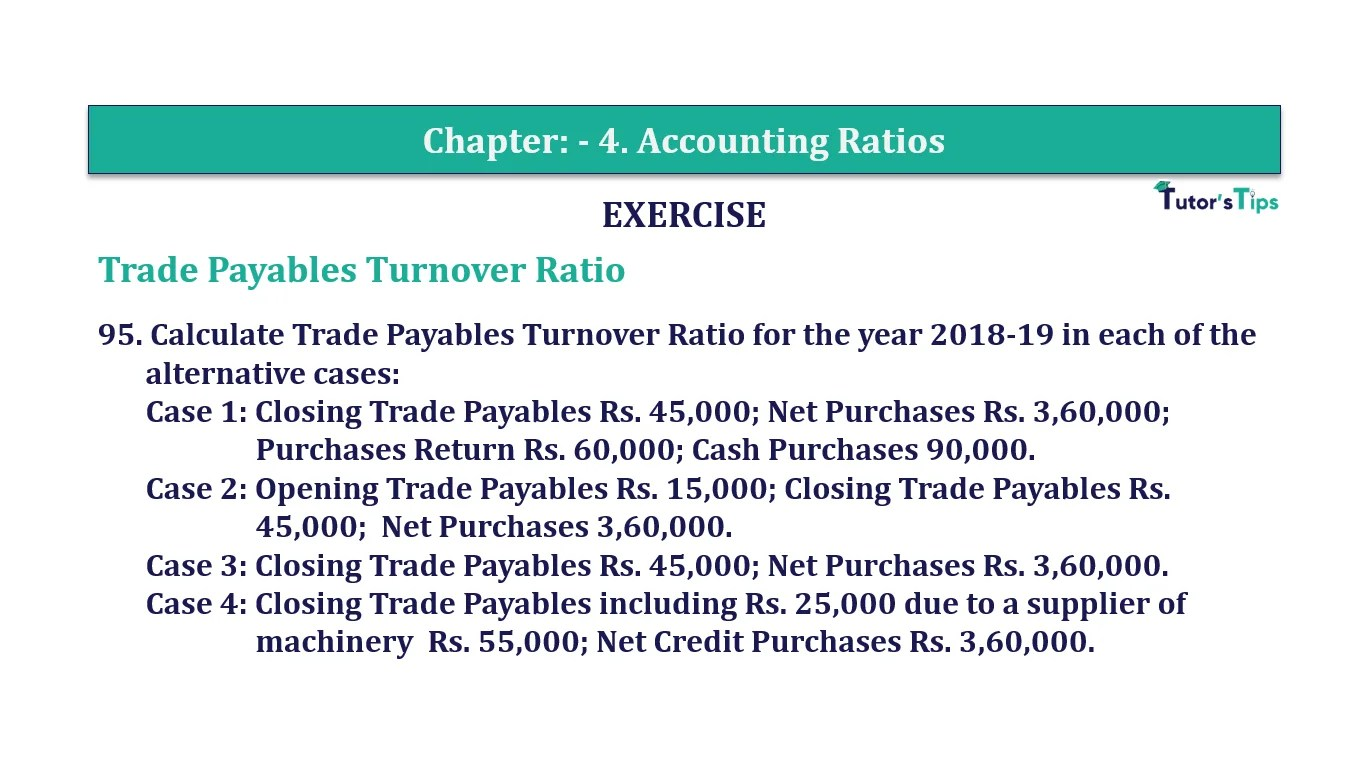# Question 95 Chapter 4 of +2-B – T.S. Grewal 12 ClassQuestion 95 Chapter 4 of +2-B

95. Calculate Trade Payables Turnover Ratio for the year 2018-19 in each of the alternative cases:
Case 1: Closing Trade Payables Rs. 45,000; Net Purchases Rs. 3,60,000;
Purchases Return Rs. 60,000; Cash Purchases 90,000.
45,000; Net Purchases 3,60,000.
Case 3: Closing Trade Payables Rs. 45,000; Net Purchases Rs. 3,60,000.
Case 4: Closing Trade Payables including Rs. 25,000 due to a supplier of
machinery Rs. 55,000; Net Credit Purchases Rs. 3,60,000.

### The solution of Question 95 Chapter 4 of +2-B: –

Case I:

 Net Credit Purchases = Net Purchases − Cash Purchases = Rs. 3,60,000 – Rs. 90,000 = Rs. 2,70,000

 = Rs. 2,70,000 Rs. 45,000 = 6 Times

Case II:

Net Credit Purchases = Rs. 3,60,000

 = Rs. 15,000 + Rs. 45,000 2 = Rs. 30,000 Trade Receivables Turnover Ratio = Net Credit Sales Average Trade Receivable = Rs. 3,60,000 Rs. 30,000 = 12 Times

Case III:

 Trade Receivables Turnover Ratio = Net Credit Sales Average Trade Receivable = Rs. 3,60,000 Rs. 45,000 = 8 Times

Case IV:

 Net Credit Payables = Trade Payables – Creditors for Machinery = Rs. 55,000 – Rs. 25,000 = Rs. 30,000

 Trade Receivables Turnover Ratio = Net Credit Sales Average Trade Receivable = Rs. 3,60,000 Rs. 30,000 = 12 Times

Balance Sheet: Meaning, Format & Examples

Comment if you have any question.

Also, Check out the solved question of previous Chapters: –